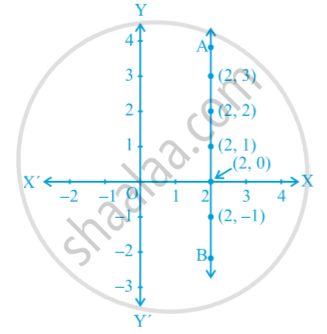# Equations of Lines Parallel to the X-axis and Y-axis

#### notes

Consider the equation x – 2 = 0. If this is treated as an equation in one variable x only, then it has the unique solution x = 2, which is a point on the number line. However, when treated as an equation in two variables, it can be expressed as x + 0 . y - 2= 0. This has infinitely many solutions. In fact , they are all of the form (2,r), where r is any real number. Also, you can check that every point of the form (2, r) is a solution of this equation. So as, an equation in two variables, x – 2 = 0 is represented by the line AB in the graph in following fig.If you would like to contribute notes or other learning material, please submit them using the button below.

### Shaalaa.com

Equations of Lines Parallel to X Axis or Y Axis [00:08:00]
S
0%